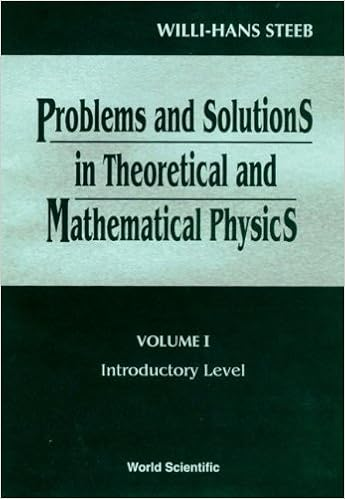## Problems and Solutions, Theoretical Mathematical Physics

Algunos libros útiles de matemáticas difíciles de conseguir.
Leonardo Sáenz
Mensajes: 2850
Registrado: Mié Jul 02, 2008 11:58 am
Verificación antispam: 4
Contactar:

### Problems and Solutions, Theoretical Mathematical PhysicsProblems and Solutions in Theoretical and Mathematical Physics , Willi-Hans Steeb
The purpose of this book is to supply a collection of problems together with their detailed solution which will prove to be valuable to students as well as to research workers in the fields of mathematics, physics, engineering and other sciences. The topics range in difficulty from elementary to advanced. Almost all problems are solved in detail and most of the problems are self-contained. All relevant definitions are given. Students can learn important principles and strategies required for problem solving. Teachers will also find this text useful as a supplement, since important concepts and techniques are developed in the problems. The material was tested in the author's lectures given around the world.
The book is divided into two volumes. Volume I presents the introductory problems for undergraduate and advanced undergraduate students. In volume II, the more advanced problems, together with their detailed solutions are collected, to meet the needs of graduate students and researchers. Problems included cover most of the new fields in theoretical and mathematical physics such as Lax representation. Bocklund transformation, soliton equations, Lie algebra valued differential forms, Hirota technique, Painleve test, the Bethe ansatz, the Yang-Baxter relation, chaos, fractals, complexity, etc.
Text contains a collection of problems with detailed solutions, for graduate students and researchers. Problems included cover most of the new fields in theoretical and mathematical physics; such as Lax representation, Backlund transformation, and soliton equations. Softcover, hardcover available.